# Blog Posts

## Previous Posts

####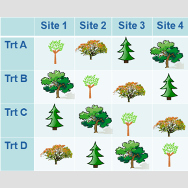What is a Completely Randomized Design?

The most basic experimental design is the completely randomized design. It is simple and straightforward when plenty of unrelated subjects are available for an experiment. It’s so simple, it almost seems obvious. But there are important principles in this simple design that are important for tackling more complex experimental designs. Let’s take a look. How […]

####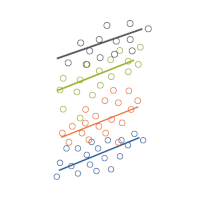Member Training: Translating Between Multilevel and Mixed Models

Data analysts can get away without ever understanding matrix algebra, certainly. But there are times when having even a basic understanding of how matrix algebra works and what it has to do with data can really make your analyses make a little more sense.

####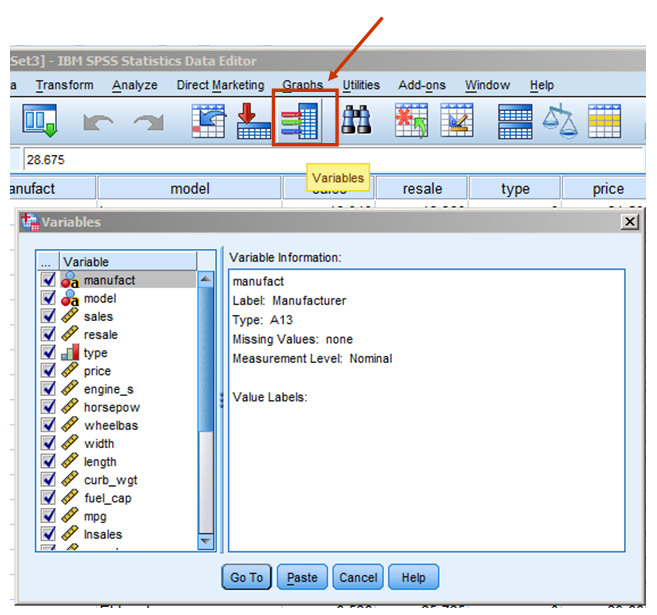Three SPSS Shortcuts that Make Life Easier

When I consult with researchers, a common part of that is going through their analysis together. Sometimes I notice that they're using some shortcut in SPSS that I had not known about. Or sometimes they could be saving themselves some headaches. So I thought I'd share three buttons you may not have noticed before that will make your data analysis more efficient.

####The Difference between Chi Square Tests of Independence and Homogeneity

A chi square test is often applied to two-way tables, like the one below. This table represents a sample of 1,322 individuals. Of these individuals, 687 are male, and 635 are female. Also 143 are union members, 159 are represented by unions, and 1,020 are not affiliated with a union. You might use a chi-square […]

####Member Training: Introduction to Stata Software Tutorial

In this 8-part tutorial, you will learn how to get started using Stata for data preparation, analysis, and graphing. This tutorial will give you the skills to start using Stata on your own. You will need a license to Stata and to have it installed before you begin.

####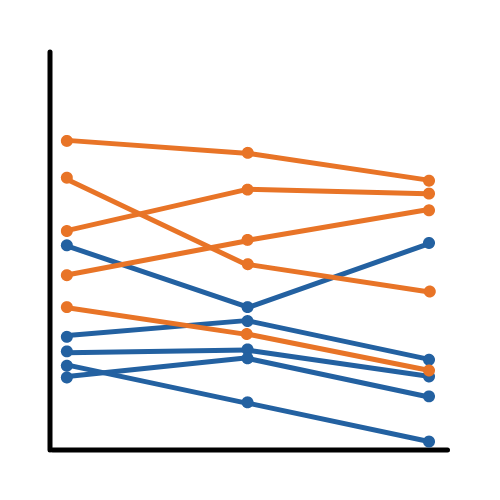An Example of Specifying Within-Subjects Factors in Repeated Measures

I want to do a GLM (repeated measures ANOVA) with the valence of some actions of my test-subjects (valence = desirability of actions) as a within-subject factor. My subjects have to rate a number of actions/behaviours in a pre-set list of 20 actions from ‘very likely to do’ to ‘will never do this’ on a scale from 1 to 7,..

####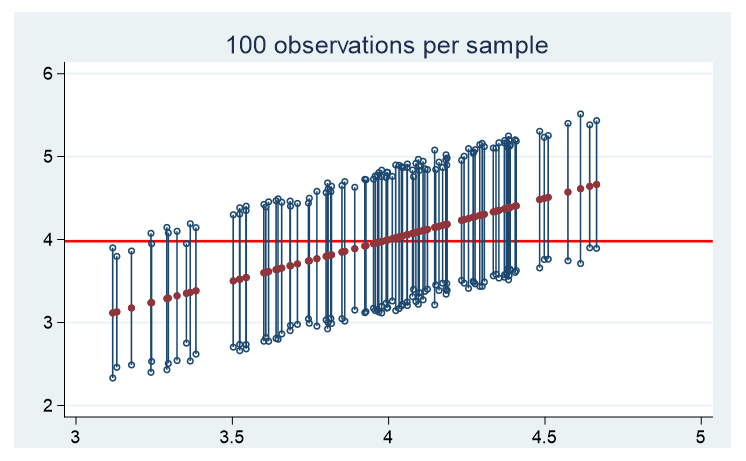How the Population Distribution Influences the Confidence Interval

Spoiler alert, real data are seldom normally distributed. How does the population distribution influence the estimate of the population mean and its confidence interval? To figure this out, we randomly draw 100 observations 100 times from three distinct populations and plot the mean and corresponding 95% confidence interval of each sample.

####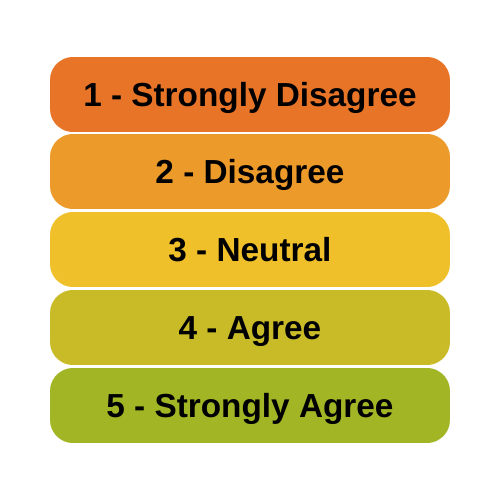Member Training: Analyzing Likert Scale Data

Is it really ok to treat Likert items as continuous? And can you just decide to combine Likert items to make a scale? Likert-type data is extremely common—and so are questions like these about how to analyze it appropriately.

####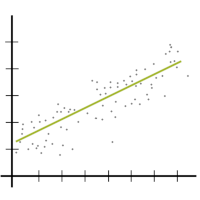The Difference Between R-squared and Adjusted R-squared

When is it important to use adjusted R-squared instead of R-squared? R², the the Coefficient of Determination, is one of the most useful and intuitive statistics we have in linear regression. It tells you how well the model predicts the outcome and has some nice properties. But it also has one big drawback.

####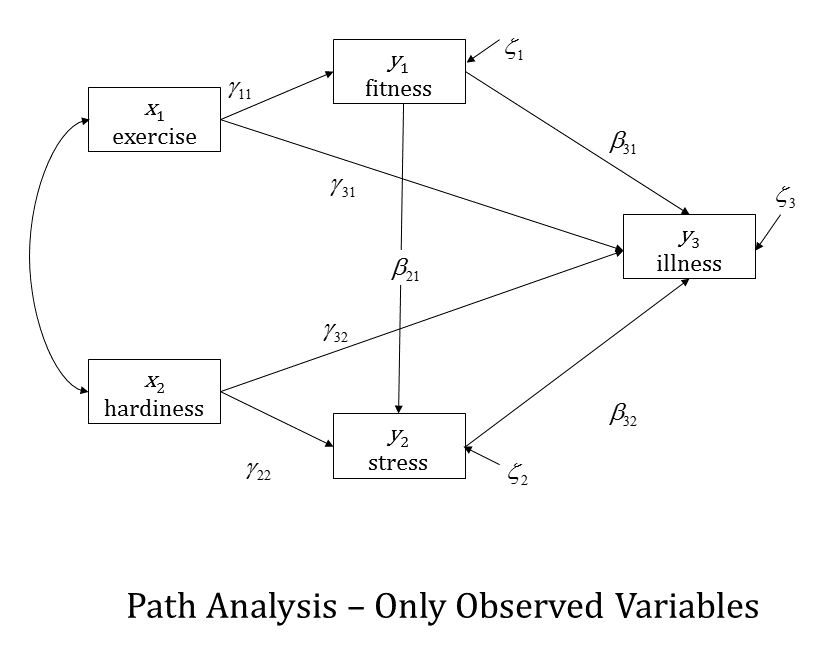The Four Models You Meet in Structural Equation Modeling

On a previous post (Why do I need to have knowledge of multiple regression to understand SEM?) we showed how a multiple regression model could be conceptualized using Structural Equation Model path diagrams. That's the simplest SEM you can create, but its real power lies in expanding on that regression model. Here I will discuss 4 ways to do that..